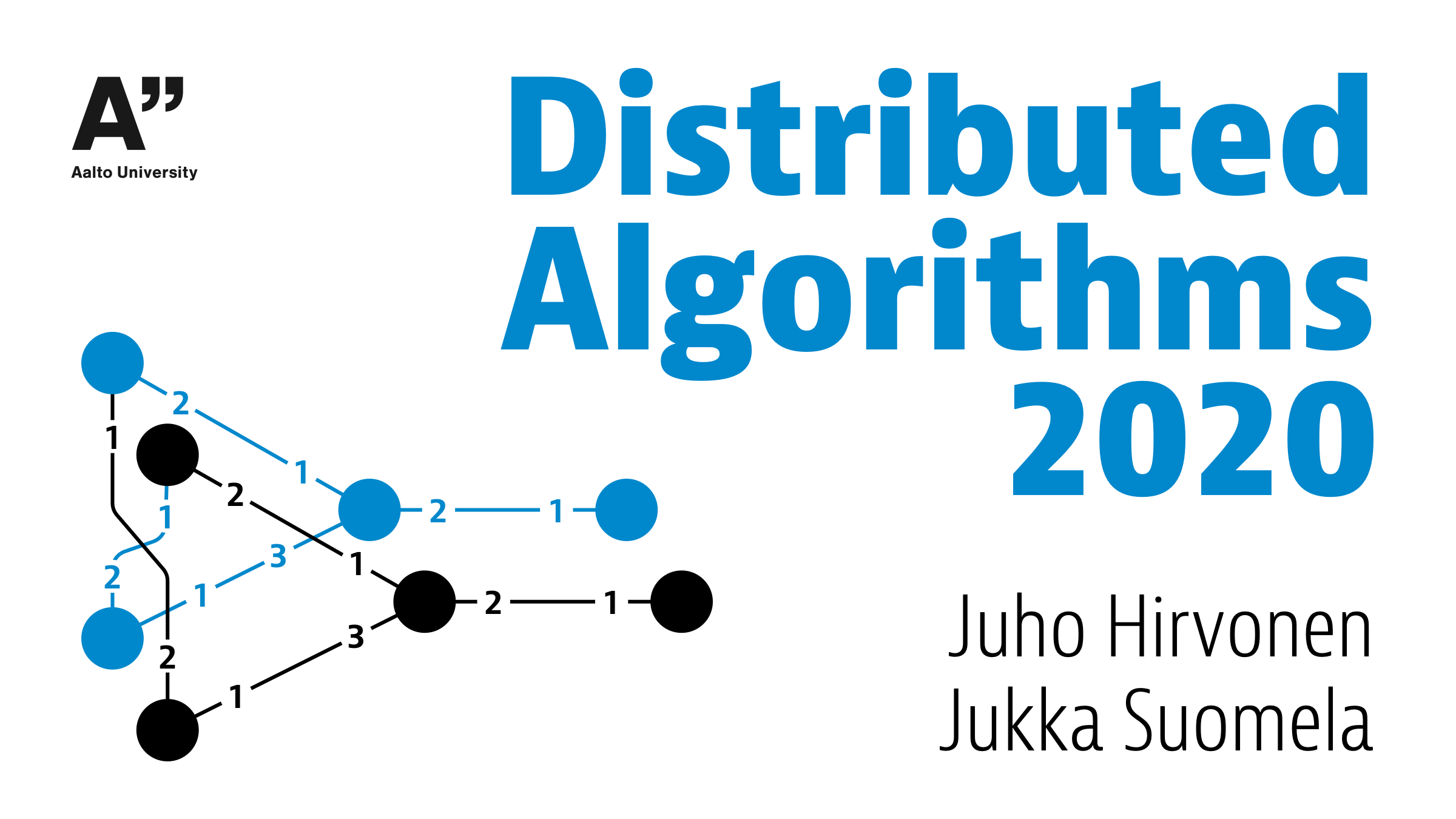Full book · PDF, 200+ pages, last updated on 2021-12-11

Source code on GitHub

This free online textbook is an introduction to the theory of distributed algorithms, with focus on distributed graph algorithms (network algorithms). The topics covered include:

• Models of computing: precisely what is a distributed algorithm, and what do we mean when we say that a distributed algorithm solves a certain computational problem?
• Algorithm design and analysis: which computational problems can be solved with distributed algorithms, which problems can be solved efficiently, and how to do it?
• Computability and computational complexity: which computational problems cannot be solved at all with distributed algorithms, which problems cannot be solved efficiently, why is this the case, and how to prove it?

No prior knowledge of distributed systems is needed. A basic knowledge of discrete mathematics and graph theory is assumed, as well as familiarity with the basic concepts from undergraduate-level courses on models on computation, computational complexity, and algorithms and data structures.